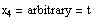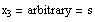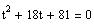Let t = r2 ==>
Char. Equation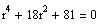Another Method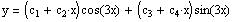The general solution is:
==> r = 3i, 3i, -3i, -3i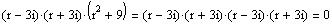Thus, the char. eqn is
cos 3x is a solution ==> 3i and -3i are roots of the characteristic equation. So, we use synthetic division to divide by 3i and -3i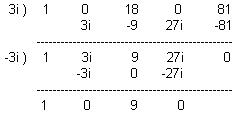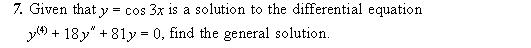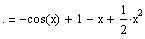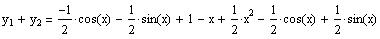Thus, the particular solution of the differential equation
y'' + y' = x + cos x + sin x is given by:
If y1 is a solution of the differential equation Ly = f(x) and y2 is a solution of the Ly = g(x) (the same diff. eqn with different non homogenous term), then y1 + y2 is a solution of Ly = f(x) + g(x).
Recall from homework problems 25 and 26 section 5.2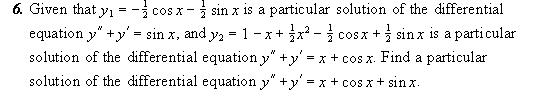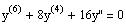And the differential equation is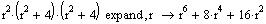The roots of the characteristic polynomial are: 0, 0, 2i, -2i, 2i, -2i

Thus, the char. poly. is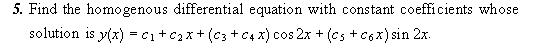Three vectors in R4 may not form a basis because the dimension of R4 is 4, which means that any basis of R4 must consist of 4 vectors.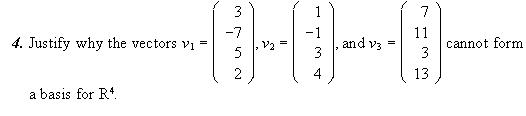Since the determinant is zero ==> the vectors are linearly dependent.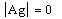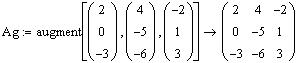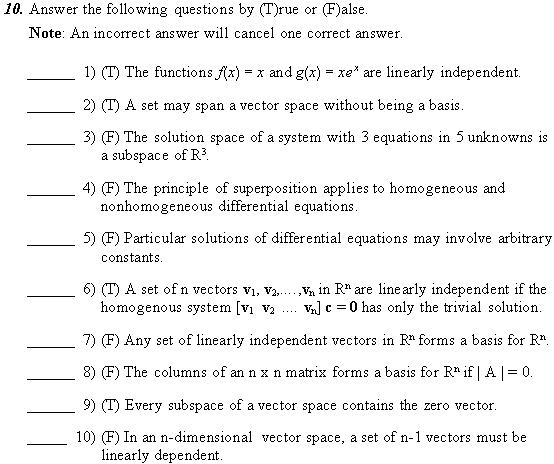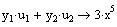Thus, the particular solution is given by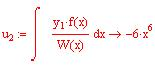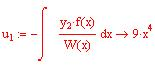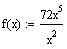Define f(x) (We must divide by x2 to put the equation in standard form)
Compute the Wronskian of y1 and y2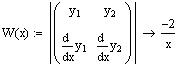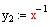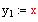We get two solutions from the complementary solution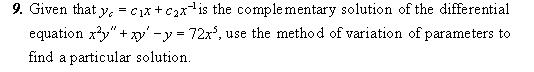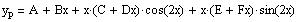But the cos 2x and sin 2x appear in the complementary solution. So, we modify yp by multiplying the cos and the sin terms by x to avoid repetition:
The form of the particular solution before modification.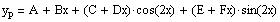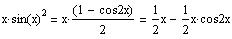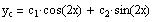==>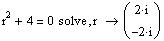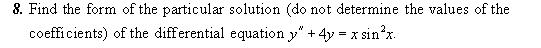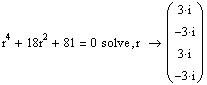r = 3i, 3i
==>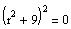==>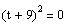==>
==>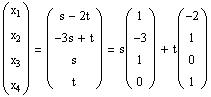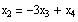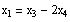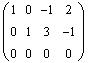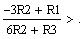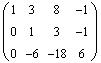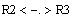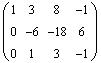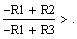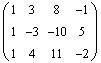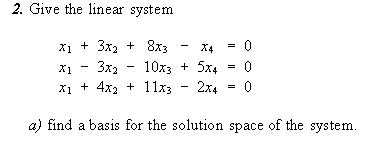Therefore, W is a subspace of R4 .
==> W is closed under scalar multiplication
cu is an element of W
==>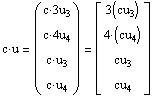Let c be an arbitrary constant.
since the first element of u+ v is 3 time the third element and the second element is 4 time the fourth element ==> u + v is an element of W ==> W is closed under addition.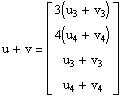==>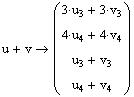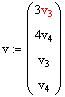and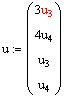Let u and v be elements of W ==>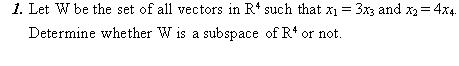May 10, 2004
Major 2 Solution
Math260-032 (A.Farhat)
Since we have 3 vectors in R3, we can find out linear dependence or independence by computing the determinant of the augmented matrix [ v1 v2 v3 ]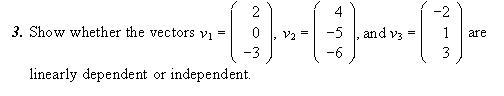The reduced row echelon form has a leading entry in the last column ==> the system has no solution ==> w can not be written as a linear combination of v1 and v2 ==> w is not a solution.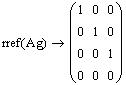Compute the reduce row echelon form of Ag.
Augment the vectors and name the matrix Ag.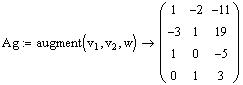Define the vector w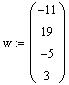This is achieved by computing the reduced row echelon form of the augmented matrix [v1 v2 w]. Mathcad will be used to do the augmentation and the reduction.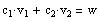We need to solve the nonhomogeneous system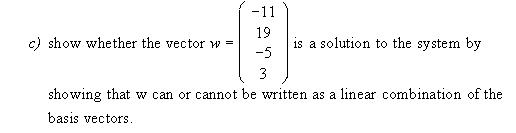The dimension of the solution space is 2 since the basis contains 2 vectors.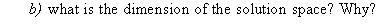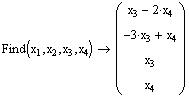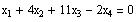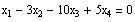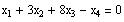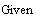We can also use the Given ... Find command to solve the system as shown below: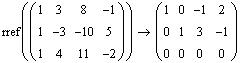Check the computation of the reduced row echelon form using Mathcad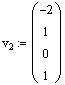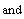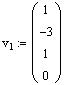Thus, the basis vectors are